SNOSB18I April   2010  – December 2014

PRODUCTION DATA.

1. Features
2. Applications
3. Description
4. Revision History
5. Pin Configuration and Functions
6. Specifications
7. Detailed Description
8. Application and Implementation
1. 8.1 Application Information
2. 8.2 Typical Application
9. Power Supply Recommendations
10. 10Layout
11. 11Device and Documentation Support
12. 12Mechanical, Packaging, and Orderable Information

• NGQ|8
• DBV|5

## 6 Specifications

### 6.1 Absolute Maximum Ratings

over operating free-air temperature range (unless otherwise noted) (1)(2)(3)
MIN MAX UNIT
Positive Supply Voltage −0.5 6.0 V
Differential Input Voltage 3 V
Input current ±10 mA
Digital Input Voltage −0.5 to VS V
Junction Temperature +150 °C
Storage Temperature (Tstg) −65 +150 °C
(1) Stresses beyond those listed under Absolute Maximum Ratings may cause permanent damage to the device. These are stress ratings only, which do not imply functional operation of the device at these or any other conditions beyond those indicated under Recommended Operating Conditions. Exposure to absolute-maximum-rated conditions for extended periods may affect device reliability.
(2) If Military/Aerospace specified devices are required, please contact the Texas Instruments Sales Office/ Distributors for availability and specifications.
(3) Electrical table values apply only for factory testing conditions at the temperature indicated. Factory testing conditions result in very limited self-heating of the device such that TJ = TA. No guarantee of parametric performance is indicated in the electrical tables under conditions of internal self-heating where TJ > TA.

### 6.2 ESD Ratings

VALUE UNIT
V(ESD) Electrostatic discharge Human-body model (HBM), per ANSI/ESDA/JEDEC JS-001(1) ±2000 V
Machine model ±200
Charged-device model (CDM), per JEDEC specification JESD22-C101(2) ±750
(1) JEDEC document JEP155 states that 500-V HBM allows safe manufacturing with a standard ESD control process. Manufacturing with less than 500-V HBM is possible with the necessary precautions. Pins listed as ±2000 V may actually have higher performance.
(2) JEDEC document JEP157 states that 250-V CDM allows safe manufacturing with a standard ESD control process. Manufacturing with less than 250-V CDM is possible with the necessary precautions. Pins listed as ±750 V may actually have higher performance.

### 6.3 Recommended Operating Conditions

over operating free-air temperature range (unless otherwise noted) (1)
MIN NOM MAX UNIT
Supply Voltage (V+ - V) 2.7 5.5 V
Operating Temperature Range −40 +125 °C

### 6.4 Thermal Information

THERMAL METRIC(1) DBV NGQ08A UNIT
5 PINS 8 PINS
RθJA Junction-to-ambient thermal resistance 179 71 °C/W

### 6.5 Electrical Characteristics 5V

The following specifications apply for single supply with VS = 5 V, RL = 100 Ω terminated to 2.5 V, gain = 10V/V, VO = 2VPP, VCM = VS/2, COMP Pin = HI (WSON-8 package), unless otherwise noted.(2)
PARAMETER TEST CONDITIONS TA = 25°C UNIT
MIN(2) TYP(3) MAX(2)
DYNAMIC PERFORMANCE
SSBW Small Signal −3dB bandwidth VO = 200 mVPP, WSON-8 package 900 MHz
VO = 200 mVPP, SOT-23-5 package 1000
AV= 4, VO = 200 mVPP,
COMP Pin = LO
800
LSBW Large signal −3dB bandwidth VO = 2VPP 380 MHz
COMP Pin = LO, AV= 4, VO = 2VPP 190
0.1 dB bandwidth AV= 10, VO = 200 mVPP, WSON-8 package 330 MHz
AV= 10, VO = 200 mVPP, SOT-23-5 package 190
AV= 4, VO = 200 mVPP,
COMP Pin = LO
95
Peaking VO = 200 mVPP, WSON-8 package 0 dB
VO = 200 mVPP, SOT-23-5 package 2
SR Slew rate AV= 10, 2 V step 1600 V/μs
AV= 4, 2 V step, COMP Pin = LO 530
tr/ tf Rise/fall time AV= 10, 2 V step, 10% to 90%,
WSON-8 package
0.90 ns
AV= 10, 2 V step, 10% to 90%,
SOT-23-5 package
0.95
AV= 4, 2 V step, 10% to 90%,
COMP Pin = LO, (Slew Rate Limited)
2.8
Ts Settling time AV= 10, 1 V step, ±0.1% 42
Overload recovery VIN = 1 VPP 2
NOISE and DISTORTION
HD2 2nd Order distortion fc = 1 MHz, VO = 2 VPP −90 dBc
COMP Pin = LO, AV= 4, fc = 1 MHz,
VO = 2 VPP
−88
fc = 10 MHz, VO = 2 VPP −70
COMP Pin = LO, fc = 10 MHz,
AV= 4 V, VO = 2 VPP
−65
HD3 3rd Order distortion fc = 1 MHz, VO = 2VPP −94 dBc
COMP Pin = LO, AV= 4,
fc = 1 MHz, VO = 2 VPP
−87
fc = 10 MHz, VO = 2 VPP −82
COMP Pin = LO, fc = 10 MHz, VO = 2VPP −75
OIP3 Two-tone 3rd order intercept point fc = 25 MHz, VO = 2 VPP composite 31 dBm
fc = 75 MHz, VO = 2 VPP composite 27
en Noise voltage Input referred f > 1MHz 0.69 nV/√Hz
in Noise current 2.6 pA/√Hz
NF Noise figure RS = RT = 50 Ω 8.0 dB
ANALOG I/O
CMVR Input voltage range CMRR > 70 dB, WSON-8 package −0.30 3.8 V
CMRR > 70 dB, SOT-23-5 package −0.30 to 3.8
VO Output voltage range RL = 100 Ω to VS/2 0.89 0.82 to 4.19 4.0 V
-40°C ≤ TJ ≤ +125°C 0.95 3.9
No Load 0.76 0.72 to 4.28 4.1
-40°C ≤ TJ ≤ +125°C 0.85 4.0
IOUT Linear output current VO = 2.5 V (1) 250 mA
VOS Input offset voltage ±150 ±780 µV
-40°C ≤ TJ ≤ +125°C ±800
TcVOS Input offset voltage temperature drift See (4) ±0.45 μV/°C
IBI Input bias current See (5) −15 −23 μA
-40°C ≤ TJ ≤ +125°C −37
IOS Input offset current ±0.1 ±1.8 μA
-40°C ≤ TJ ≤ +125°C ±3.0
TCIOS Input offset voltage temperature drift See (4) ±2.8 nA/°C
CCM Input capacitance Common Mode 1.7 pF
CDIFF Differential Mode(6) 4
RCM Input resistance Common Mode 450
MISCELLANEOUS PARAMETERS
CMRR Common mode rejection ratio VCM from 0 V to 3.7 V, WSON-8 package 82 87 dB
-40°C ≤ TJ ≤ +125°C 70
VCM from 0 V to 3.7 V, SOT-23-5 package 87
PSRR Power supply rejection ratio 81 83
-40°C ≤ TJ ≤ +125°C 78
AVOL Open loop gain WSON-8 package 74 78
-40°C ≤ TJ ≤ +125°C 72
SOT-23-5 package 78
DIGITAL INPUTS/TIMING
VIL Logic low-voltage threshold PD and COMP pins, WSON-8 package 0.8 V
VIH Logic high-voltage threshold PD and COMP pins, WSON-8 package 2.5
IIL Logic Low-bias current PD and COMP pins = 0.8 V, WSON-8 package(5) −23 −28 −34 µA
-40°C ≤ TJ ≤ +125°C −19 −38
IIH Logic High-bias current PD and COMP pins = 2.5 V, WSON-8 package(5) −16 −22 −27
-40°C ≤ TJ ≤ +125°C −14 −29
Ten Enable time WSON-8 package 75 ns
Tdis Disable time 80
POWER REQUIREMENTS
IS Supply current No Load, Normal Operation (PD Pin = HI or open for WSON-8 package) 15.5 16.7 mA
-40°C ≤ TJ ≤ +125°C 18.2
No Load, Shutdown (PD Pin =LO for WSON-8 package) 1.1 1.85
-40°C ≤ TJ ≤ +125°C 2.0
(1) The maximum continuous output current (IOUT) is determined by device power dissipation limitations. Continuous short circuit operation at elevated ambient temperature can result in exceeding the maximum allowed junction temperature of 150°C
(2) All limits are ensured by testing or statistical analysis
(3) Typical numbers are the most likely parametric norm.
(4) Drift determined by dividing the change in parameter at temperature extremes by the total temperature change.
(5) Negative input current implies current flowing out of the device
(6) Simulation results.

### 6.6 Electrical Characteristics 3.3V

The following specifications apply for single supply with VS = 3.3 V, RL = 100 Ω terminated to 1.65 V, gain = 10V/V, VO = 1 VPP, VCM = VS/2, COMP Pin = HI (WSON-8 package), unless otherwise noted.(2)
PARAMETER TEST CONDITIONS TA = 25°C UNIT
MIN(4) TYP(5) MAX(4)
DYNAMIC PERFORMANCE
SSBW Small signal −3dB bandwidth VO = 200 mVPP, WSON-8 package 820 MHz
VO = 200 mVPP, SOT-23-5 package 950
COMP Pin = LO, AV= 4,
VO = 200 mVPP
730
LSBW Large signal −3dB bandwidth VO = 1VPP 540 MHz
COMP Pin = LO, AV= 4, VO = 1VPP 320
0.1 dB Bandwidth AV= 10, VO = 200 mVPP,
WSON-8 package
330 MHz
AV= 10, VO = 200 mVPP,
SOT-23-5 package
190
COMP Pin = LO, AV= 4,
VO = 200 mVPP
85
Peaking VO = 200 mVPP, WSON-8 package 0 dB
VO = 200 mVPP, SOT-23-5 package 1.8
SR Slew rate AV= 10, 1.3V step 1100 V/µs
COMP Pin = LO, AV= 4, 1.3V step 500
tr/ tf Rise/fall time AV= 10, 1V step, 10% to 90%,
WSON-8 package
0.7 ns
AV= 10, 1V step, 10% to 90%,
SOT-23-5 package
0.55
AV= 4, COMP Pin = LO, 1V step, 10% to 90% (Slew Rate Limited) 1.3
Ts Settling time AV= 10, 1V step, ±0.1% 70
Overload recovery VIN = 1VPP 2
NOISE and DISTORTION
HD2 2nd Order distortion fc = 1MHz, VO = 1VPP -82 dBc
COMP Pin = LO, AV= 4,
fc = 1MHz, VO = 1VPP
-88
fc = 10 MHz, VO = 1VPP -67
COMP Pin = LO, fc = 10 MHz,
AV= 4V, VO = 1VPP
-74
HD3 3rd Order distortion fc = 1MHz, VO = 1VPP -94 dBc
COMP Pin = LO, AV= 4,
fc = 1MHz, VO = 1VPP
-112
fc = 10 MHz, VO = 1VPP -79
COMP pin = LO, fc = 10 MHz,
VO = 1VPP
-96
OIP3 Two-tone 3rd order intercept point fc = 25 MHz, VO = 1VPP composite 30 dBm
fc = 75 MHz, VO = 1VPP composite 26
en Noise voltage Input referred, f > 1MHz 0.69 nV/√Hz
in Noise current 2.6 pA/√Hz
NF Noise figure RS = RT = 50 Ω 8.0 dB
ANALOG I/O
CMVR Input voltage range CMRR > 70 dB, WSON-8 package -0.30 2.1 V
CMRR > 70 dB, SOT-23-5 package -0.30 to 2.1
VO Output voltage range RL = 100 Ω to VS/2 0.90 0.79 to 2.50 2.4
-40°C ≤ TJ ≤ +125°C 0.95 2.3
No load 0.76 0.70 to 2.60 2.5
-40°C ≤ TJ ≤ +125°C 0.80 2.4
IOUT Linear output current VO = 1.65 V(3) 230 mA
VOS Input offset voltage ±150 ±680 µV
-40°C ≤ TJ ≤ +125°C ±700
TcVOS Input offset voltage temperature drift See (6) ±1 µV/°C
IBI Input bias current See (7) -15 -23 µA
-40°C ≤ TJ ≤ +125°C -35
IOS Input offset current ±0.13 ±1.8
-40°C ≤ TJ ≤ +125°C ±3.0
TCIOS Input offset voltage temperature drift See (6) ±3.2 nA/°C
CCM Input capacitance Common Mode 1.7 pF
CDIFF Differential Mode(8) 4
RCM Input resistance Common Mode 1
MISCELLANEOUS PARAMETERS
CMRR Common mode rejection ratio VCM from 0 V to 2.0 V, WSON-8 package 84 87 dB
-40°C ≤ TJ ≤ +125°C 81
VCM from 0 V to 2.0 V,
SOT-23-5 package
87
PSRR Power supply rejection ratio 82 84
-40°C ≤ TJ ≤ +125°C 79
AVOL Open loop gain WSON-8 package 78 79
-40°C ≤ TJ ≤ +125°C 73
SOT-23-5 package 79
DIGITAL INPUTS/TIMING
VIL Logic low-voltage threshold PD and COMP pins, WSON-8 package 0.8 V
VIH Logic high-voltage threshold 2.0
IIL Logic low-bias current PD and COMP pins = 0.8 V,
WSON-8 package(7)
-17 -23 -28 µA
-40°C ≤ TJ ≤ +125°C -14 -32
IIH Logic high-bias current PD and COMP pins = 2.0 V, WSON-8 package(7) -16 -22 -27
-40°C ≤ TJ ≤ +125°C -13 -31
Ten Enable time WSON-8 package 75 ns
Tdis Disable time 80
POWER REQUIREMENTS
IS Supply current No Load, Normal Operation (PD Pin = HI or open for WSON-8 package) 13.7 14.9 mA
-40°C ≤ TJ ≤ +125°C 16.0
No Load, Shutdown (PD Pin = LO for WSON-8 package) 0.89 1.4
-40°C ≤ TJ ≤ +125°C 1.5
(1) Absolute maximum ratings indicate limits beyond which damage to the device may occur. Operating Ratings indicate conditions for which the device is intended to be functional, but specific performance is not ensured. For ensured specifications and the test conditions, see the Electrical Characteristics.
(2) Electrical table values apply only for factory testing conditions at the temperature indicated. Factory testing conditions result in very limited self-heating of the device such that TJ = TA. No guarantee of parametric performance is indicated in the electrical tables under conditions of internal self-heating where TJ > TA.
(3) The maximum continuous output current (IOUT) is determined by device power dissipation limitations. Continuous short circuit operation at elevated ambient temperature can result in exceeding the maximum allowed junction temperature of 150°C
(4) All limits are ensured by testing or statistical analysis.
(5) Typical numbers are the most likely parametric norm.
(6) Drift determined by dividing the change in parameter at temperature extremes by the total temperature change.
(7) Negative input current implies current flowing out of the device.
(8) Simulation results.

### 6.7 Typical Performance Characteristics

Unless otherwise specified, VS = ±2.5V, Rf = 240 Ω, RL = 100 Ω, VO = 2 VPP, COMP pin = HI, AV = +10 V/V, WSON-8 and SOT-23-5 packages (unless specifically noted).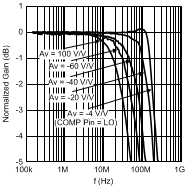Vo = 2 Vpp
Figure 1. Inverting Frequency Response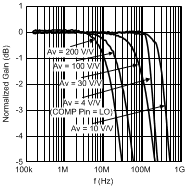Vo = 2 Vpp
Figure 3. Non-Inverting Frequency ResponseVo = 0.2 Vpp
Figure 5. Non-Inverting Frequency Response,
WSON-8 Package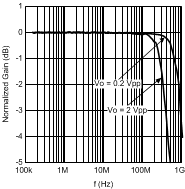Av = 10 V/V
Figure 7. Non-Inverting Frequency Response
with Varying VO, WSON-8 Package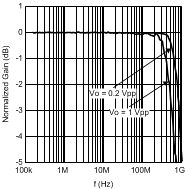Av = 10 V/V Vs = +/-1.5 V
Figure 9. Non-Inverting Frequency Response
with Varying VO, WSON-8 Package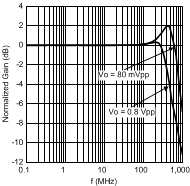Av = 4 V/V COMP Pin = LO
Figure 11. Non-Inverting Frequency Response
with Varying VO, WSON-8 Package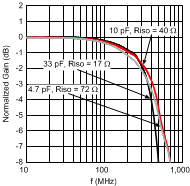RL = 100 Ω || CL RISO as noted (measured @ CL)Figure 15. Frequency Response vs. Rf, WSON-8 Package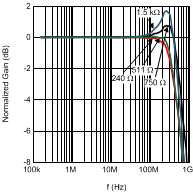VS = ±1.5 V VO = 1 VPP
Figure 17. Frequency Response vs. Rf, WSON-8 Package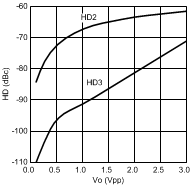20 MHz
Figure 19. Distortion vs. Swing, WSON-8 Package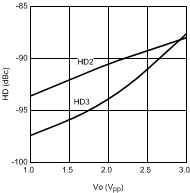1 MHz
Figure 21. Distortion vs. Swing, WSON-8 Package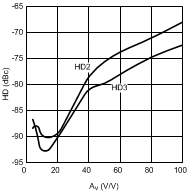1 MHz Vout = 2 Vpp
Figure 23. Distortion vs. Gain, WSON-8 Package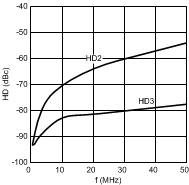Vo = 2 Vpp
Figure 25. Distortion vs. Frequency, WSON-8 Package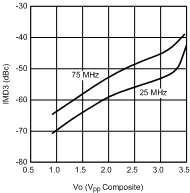Figure 27. 3rd Order Intermodulation Distortion
vs. Output VoltageFigure 29. Input Noise Current vs. Frequency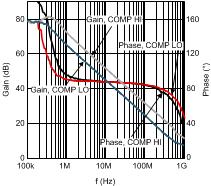Figure 31. Open Loop Gain/Phase Response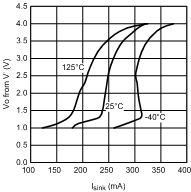Figure 33. Output Sink Current WSON-8 Package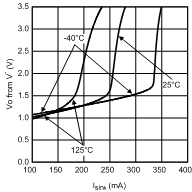Figure 35. Output Sink Current, SOT-23-5 Package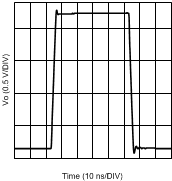AV= 4V/V COMP Pin = LO
Figure 37. Large Signal Step Response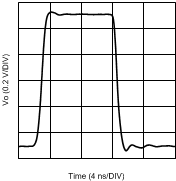Vs = 3.3 V
Figure 39. Large Signal Step Response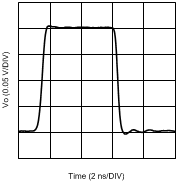Vs = 3.3 V
Figure 41. Small Signal Step Response, WSON-8 Package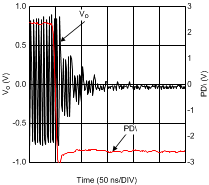Figure 43. Turn-Off Waveform, WSON-8 Package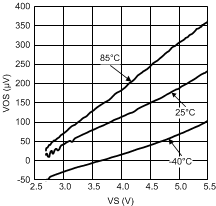Figure 45. Offset Voltage vs. Supply Voltage
(Typical Unit)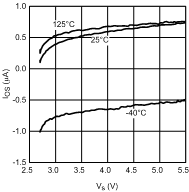Figure 47. Input Offset Current vs. Supply Voltage (Typical Unit)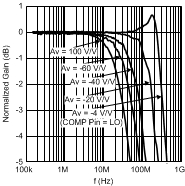Vo = 1 Vpp Vs = ±1.5V
Figure 2. Inverting Frequency Response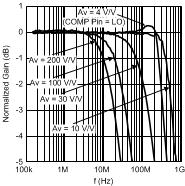Vo = 1 Vpp Vs = ±1.5 V
Figure 4. Non-Inverting Frequency Response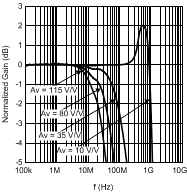Vo = 0.2 Vpp
Figure 6. Non-Inverting Frequency Response,
SOT-23-5 Package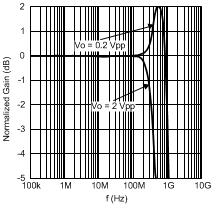Av=10 V/V
Figure 8. Non-Inverting Frequency Response
with Varying VO, SOT-23-5 PackageAv=10 V/V Vs= +/-1.5 V
Figure 10. Non-Inverting Frequency Response
with Varying VO, SOT-23-5 Package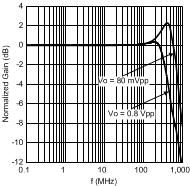Av = 4 V/V Vs = ±1.5 V COMP Pin = LO
Figure 12. Non-Inverting Frequency Response
with Varying VO, WSON-8 Package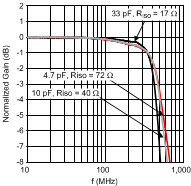RL = 100 Ω || CL, AV = 4 V/V COMP Pin = LO RISO as noted (measured @ CL)
WSON-8 Package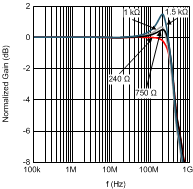Figure 16. Frequency Response vs. Rf, SOT-23-5 Package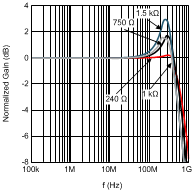VS = ± 1.5 V VO = 1 VPP
Figure 18. Frequency Response vs. Rf, SOT-23-5 Package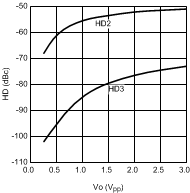20 MHz
Figure 20. Distortion vs. Swing, SOT-23-5 Package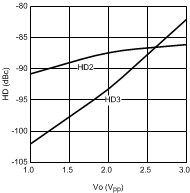1 MHz
Figure 22. Distortion vs. Swing, SOT-23-5 Package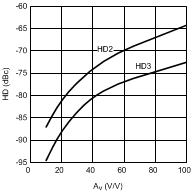1 MHz Vout = 2 Vpp
Figure 24. Distortion vs. Gain, SOT-23-5 Package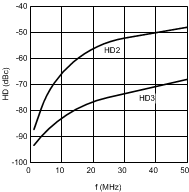Vo = 2 Vpp
Figure 26. Distortion vs. Frequency, SOT-23-5 Package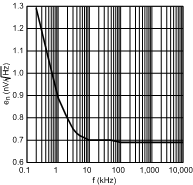Figure 28. Input Noise Voltage vs. Frequency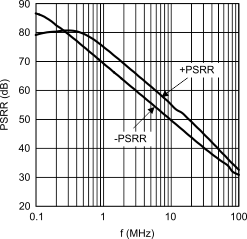Figure 30. PSRR vs. Frequency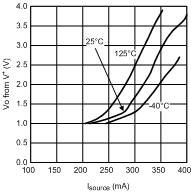Figure 32. Output Source Current, WSON-8 PackageFigure 34. Output Source Current, SOT-23-5 PackageFigure 36. Large Signal Step Response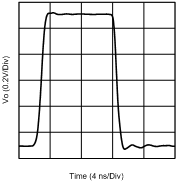Figure 38. Large Signal Step Response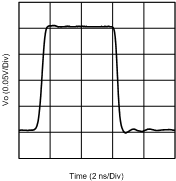Figure 40. Small Signal Step Response, WSON-8 Package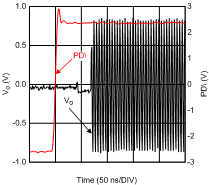Figure 42. Turn-On Waveform, WSON-8 Package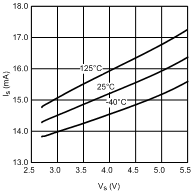Figure 44. Supply Current vs. Supply VoltageFigure 46. Input Bias Current vs. Supply Voltage
(Typical Unit)